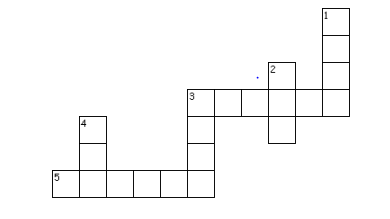# Data Handling Important Questions

In this page we have Data Handling Important Questions for Class 7 Maths Chapter 3 . Hope you like them and do not forget to like , social share and comment at the end of the page.
Question 1.
Fill in the blanks:
a. Different forms of central tendency are ________________and__________
b. The difference between the highest and lowest values of observations in a set of data is called the_____________
c. On dividing the sum of observation by total number of observations, we obtain the________
d. The representation of numbers using bars of uniform width, the length of the bars depicting the frequency is called the____________
e. The possible results of an experiment are called______________
f. When the raw data is arranged in ascending or descending order, it forms an__________
g. The measure of chance of happening something is called________________
h. The probability of getting 1 in tossing of coin is  _______________
i. The probability of getting a tail in a throw of a dice is________________.
j. The outcomes which have equal chances of occurrence are called_____________.
k. The number that divides the given data into two equal parts after arranging it in ascending or descending order is called the__________________
l. The observation that occurs most often is called___________
m. Probability of getting 7 when a number from 1 to 6 is selected is__________.
n. Probability of an event always lie between ____________and___________.
o. If the arithmetic means of 8, 4, x, 6, 2, 7 is 5, then the value of x is _________
p. The median of the data: 3, 4, 5, 6, 7, 3, 4 is    _______

Question 2.
State true of false.
i. Mean of a data set is the value which divides the value into two equal parts.
ii. A data set may have more than one mode.
iii. The value of median changes if the value in a data set are arranged in ascending order instead of descending order.
iv. The data set 4, 8, 11, 7, 5, 0 has the mode 0.
v. Rolling the number 7 on a standard die is an impossible event.
vi. The median can be value which is not equal to any of the observation.
vii.  Mean of the data is always from the given data.
viii. It is impossible to get a sum of 14 of the numbers on both dice when a pair of dice is thrown together
ix. Mode of the data is always from the given data.
x. Mean of the observations can be lesser than each of the observations.

Question 3.
The mean of 5 numbers is 25. If the four numbers 16, 26, 31, 32. Find the fifth numbers.

Question 4Across
3. _____gives the middle observation of a given data
5. A _______ bar graph helps in comparing two collections of data at a glance.
Down
1. It is defined as sum of all observations divided by total number of observations
2. Throwing a die gives ____ possible outcomes.
3. The ______ of a set of observations is the observation that occurs most often
4. Tossing a coin gives ___ outcomes

Question 5
A batsman scored the following number of runs in six innings:
36, 35, 50, 46, 60, 55
Calculate the mean runs scored by him in an inning

1.
a. Mean, median and Mode
b. Range
c. mean
d. Bar graph
e. outcomes
f. array
g. probability
h. 0
i. 0
j. equally likely
k. median
l. mode
m.  0
n. 0 and 1
o.  3
p. 4

2.
a. False
b. True
c.  false
d.  False
e. True
f. false
g. false
h.  True
i. True
j. False

3. 20
4.
i. Mean
ii. six
iii. Mode
median
iv. two
v. double
5. 47